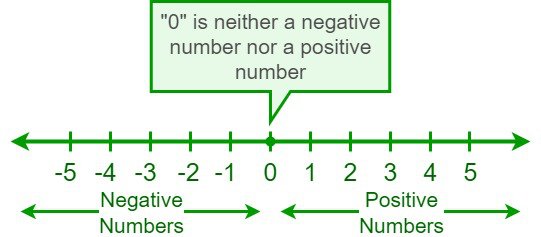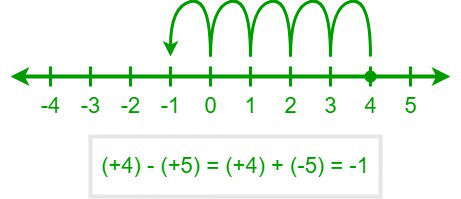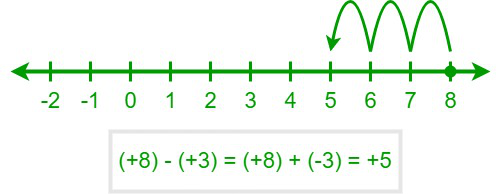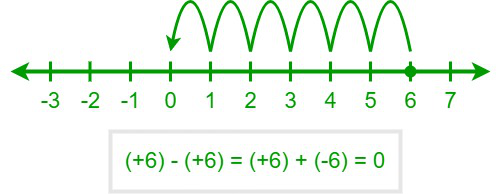Open in App
Not now

# What is the rule for subtracting positive numbers?

• Last Updated : 12 Jul, 2022

The branch of mathematics that deals with arithmetic operations and the symbols that represent them is referred to as algebra. Algebra covers a wide range of topics, from finding solutions to simple equations to studying abstractions. Algebra aids in the solution of mathematical equations and allows one to calculate unknown quantities such as proportions, the speed of a boat, and percentages. In algebra, the entities are represented by letters or other symbols to build a mathematical equation. In our everyday lives, using algebraic formulae, we can determine the distance between two places, the capacity of containers, and discounts when they are required.

### Positive Numbers

Numbers play a crucial role in every aspect of our lives, from keeping things organized to counting the items on a list, from preparing every month’s budget to calculating the monthly expenses, and much more. There are various kinds of numbers, namely positive numbers, negative numbers, even numbers, odd numbers, prime numbers, composite numbers, consecutive numbers, perfect numbers, etc. Numbers are categorized into various types based on their properties, and a negative number is one of them. A positive number can be written as being preceded by the (+) symbol or can be simply a number and its value is always greater than zero. Positive numbers are displayed on the right side of the zero on a number line. 2, 15, 23.86, 17/19, etc. are some examples of positive numbers, i.e., positive numbers may be integers, decimals, or fractions.Number Line

### Rules for Subtracting Positive Numbers

Specific guidelines must be followed when we perform arithmetic operations like addition, subtraction, multiplication, and division on numbers.

The rule for Subtraction

A positive number is subtracted from another by changing its sign, which is followed by adding the sign-changed number to the first number. However, the final output may be a positive or negative number and the magnitude of the result will be less than both operands if none of the operands is zero.

Case 1: Second operand > the first operand

The final output will be a negative number if the magnitude of the second operand is greater than the first operand. For example, (+4) – (+5) will be equal to (+4) + (-5). We know that, on a number line, we shift to the left to add a negative number. So, as “–5” is added to (+4), we shift 5 units to the left, starting from (+4). Hence, the answer is “–1.”Case 2: Second operand < the first operand

The final output will be a positive number if the magnitude of the second operand is less than the first operand. For example, +8 – (+3) will be equal to (+8) + (–3). We know that, on a number line, we shift to the left to add a negative number. So, as “–3” is added to (+8), we shift 3 units to the left, starting from (+8). Hence, the answer is “+5.”Case 3: Second operand = the first operand

The final output will be zero if the magnitude of the second operand is equal to the first operand. For example, (+6) – (+6) will be equal to (+6) + (–6). We know that, on a number line, we shift to the left to add a negative number. So, as “–6” is added to (+6), we shift 6 units to the left, starting from (+6). Hence, the answer is “0.”### Sample Problems

Problem 1: Determine the solution for: (3 × 4) – (2 × 5) – (1 × 8).

Solution:

Given,

(3 × 4) – (2 × 5) – (1 × 8)

By solving the bracket at first, we get

= (12) – (10) – (8)

Now, open the brackets.

= 12 – 10 – 8

Now, add the positive and negative integers separately.

= 12 – 18

= -6

Therefore, (3 × 4) – (2 × 5) – (1 × 8) = -6

Problem 2:  Subtract  6a2 + 8b2 + 20ab from (3a + 5b)2.

Solution:

Given,

(3a – 5b)2 – (6a2 + 8b2 + 20ab)

= (9a2 + 25b2+ 30ab) – (6a2 + 8b2 + 20ab) {Since, (a + b)2 = a2 + b2 + 2ab}

Now, open the brackets,

= 9a2 + 25b2 + 30ab – 6a2 – 8b2 – 20ab

Now, add or subtract like terms.

= 9a2 – 6a2 + 25b2 – 8b2 + 30ab – 20ab

= 3a2 + 17b2 + 10ab

Hence, (3a + 5b)2 – (6a2 + 8b2 + 20ab) = 3a2 + 17b2 + 10ab

Problem 3: Evaluate: 68 – 35 – 10 – 43 + 14.

Solution:

Given,

68 – 35 – 10 – 43 + 14

Add the positive and negative integers separately

= 68 + 14 – 35 – 10 – 43

= 82 – 88

= (+82) + (-88) = -6

Hence, [68 – 35 – 10 – 43 + 14] = -6

Problem 4: Subtract the given positive integers,

• Subtract 10 from 5
• Subtract 17 from 29
• Subtract 56 from 87

Solution:

• Subtract 10 from 5 = (+5) – (+10)

From the rule of subtracting negative numbers, we know that, when a positive number is subtracted from another by changing its sign, which is followed by adding the sign-changed number to the first number.

Hence, (+5) – (+10) = (+5) + (–10) = –5

• Subtract 17 from 29 = (+29) – (+17)

= (+29) + (–17) = +12

• Subtract 56 from 87 = (+87) – (+56)

= (+87) + (–56) = +31

Problem 5: Subtract (5x + 2y)2  from (7x + 6y)2.

Solution:

Given,

(7x + 6y)2 – (5x + 2y)2

=  (49x2 + 36y2 + 84xy) – (25x2 + 4y2 + 20xy)       {Since, (a + b)2 = a2 + b2 + 2ab}

Now, open the brackets,

= 49x2 + 36y2 + 84xy- 25x2 – 4y2 – 20xy

Now, add or subtract like terms,

=  49x2 – 25x2 + 36y2 – 4y2 +84xy – 20xy

= 24x2 + 32y2 + 64xy

Hence, (7x + 6y)2 – (5x + 2y)2 = 24x2 + 32y2 + 64xy

Problem 6: Determine the solution for: (48 ÷ 3) – (6 × 7) – (12 × 5).

Solution:

Given,

(48 ÷ 3) – (6 × 7) – (12 × 5)

By solving the bracket at first, we get

= (16) – (42) – (60)

Now, open the brackets.

= 16 – 42 – 60

Now, add the positive and negative integers separately.

= 16 – 102

= -86

Therefore, (48 ÷ 3) – (6 × 7) – (12 × 5) = -86.

My Personal Notes arrow_drop_up
Related Articles